Plots a ggplot2 object in 3D by mapping the color or fill aesthetic to elevation.

Currently, this function does not transform lines mapped to color into 3D.

If there are multiple legends/guides due to multiple aesthetics being mapped (e.g. color and shape), the package author recommends that the user pass the order of the guides manually using the ggplot2 function "guides(). Otherwise, the order may change when processing the ggplot2 object and result in a mismatch between the 3D mapping and the underlying plot.

Using the shape aesthetic with more than three groups is not recommended, unless the user passes in custom, solid shapes. By default in ggplot2, only the first three shapes are solid, which is a requirement to be projected into 3D.

plot_gg(
ggobj,
width = 3,
height = 3,
height_aes = NULL,
invert = FALSE,
units = c("in", "cm", "mm"),
scale = 150,
pointcontract = 0.7,
offset_edges = FALSE,
preview = FALSE,
raytrace = TRUE,
sunangle = 315,
anglebreaks = seq(30, 40, 0.1),
multicore = FALSE,
lambert = TRUE,
triangulate = TRUE,
max_error = 0.001,
max_tri = 0,
verbose = FALSE,
emboss_text = 0,
emboss_grid = 0,
reduce_size = NULL,
save_height_matrix = FALSE,
...
)

## Arguments

ggobj

ggplot object to projected into 3D.

width

Default 3. Width of ggplot, in units.

height

Default 3. Height of ggplot, in units.

height_aes

Default NULL. Whether the fill or color aesthetic should be used for height values, which the user can specify by passing either fill or color to this argument. Automatically detected. If both fill and color aesthetics are present, then fill is default.

invert

Default FALSE. If TRUE, the height mapping is inverted.

Default 0.5. The intensity of the calculated shadows.

units

Default in. One of c("in", "cm", "mm").

scale

Default 150. Multiplier for vertical scaling: a higher number increases the height of the 3D transformation.

pointcontract

Default 0.7. This multiplies the size of the points and shrinks them around their center in the 3D surface mapping. Decrease this to reduce color bleed on edges, and set to 1 to turn off entirely. Note: If size is passed as an aesthetic to the same geom that is being mapped to elevation, this scaling will not be applied. If alpha varies on the variable being mapped, you may want to set this to 1, since the points now have a non-zero width stroke outline (however, mapping alpha in the same variable you are projecting to height is probably not a good choice. as the alpha variable is ignored when performing the 3D projection).

offset_edges

Default FALSE. If TRUE, inserts a small amount of space between polygons for "geom_sf", "geom_tile", "geom_hex", and "geom_polygon" layers. If you pass in a number, the space between polygons will be a line of that width. You can also specify a number to control the thickness of the offset. Note: this feature may end up removing thin polygons from the plot entirely--use with care.

preview

Default FALSE. If TRUE, the raytraced 2D ggplot will be displayed on the current device.

raytrace

Default FALSE. Whether to add a raytraced layer.

sunangle

Default 315 (NW). If raytracing, the angle (in degrees) around the matrix from which the light originates.

anglebreaks

Default seq(30,40,0.1). The azimuth angle(s), in degrees, as measured from the horizon from which the light originates.

multicore

Default FALSE. If raytracing and TRUE, multiple cores will be used to compute the shadow matrix. By default, this uses all cores available, unless the user has set options("cores") in which the multicore option will only use that many cores.

lambert

Default TRUE. If raytracing, changes the intensity of the light at each point based proportional to the dot product of the ray direction and the surface normal at that point. Zeros out all values directed away from the ray.

triangulate

Default FALSE. Reduce the size of the 3D model by triangulating the height map. Set this to TRUE if generating the model is slow, or moving it is choppy. Will also reduce the size of 3D models saved to disk.

max_error

Default 0.001. Maximum allowable error when triangulating the height map, when triangulate = TRUE. Increase this if you encounter problems with 3D performance, want to decrease render time with render_highquality(), or need to save a smaller 3D OBJ file to disk with save_obj(),

max_tri

Default 0, which turns this setting off and uses max_error. Maximum number of triangles allowed with triangulating the height map, when triangulate = TRUE. Increase this if you encounter problems with 3D performance, want to decrease render time with render_highquality(), or need to save a smaller 3D OBJ file to disk with save_obj(),

verbose

Default TRUE, if interactive(). Prints information about the mesh triangulation if triangulate = TRUE.

emboss_text

Default 0, max 1. Amount to emboss the text, where 1 is the tallest feature in the scene.

emboss_grid

Default 0, max 1. Amount to emboss the grid lines, where 1 is the tallest feature in the scene. By default, the minor grid lines will be half the size of the major lines. Pass a length-2 vector to specify them seperately (second value is the minor grid height).

reduce_size

Default NULL. A number between 0 and 1 that specifies how much to reduce the resolution of the plot, for faster plotting. By default, this just decreases the size of height map, not the image. If you wish the image to be reduced in resolution as well, pass a numeric vector of size 2.

save_height_matrix

Default FALSE. If TRUE, the function will return the height matrix used for the ggplot.

Default FALSE. If TRUE, the function will return the shadow matrix for use in future updates via the shadow_cache argument passed to ray_shade.

Default NULL. A cached shadow matrix (saved by the a previous invocation of plot_gg(..., save_shadow_matrix=TRUE) to use instead of raytracing a shadow map each time.

...

Additional arguments to be passed to plot_3d().

## Value

Opens a 3D plot in rgl.

## Examples

library(ggplot2)
library(viridis)
# \dontshow{
options("cores"=2)
# }

ggdiamonds = ggplot(diamonds, aes(x, depth)) +
stat_density_2d(aes(fill = stat(nlevel)), geom = "polygon", n = 200, bins = 50,contour = TRUE) +
facet_wrap(clarity~.) +
scale_fill_viridis_c(option = "A")
# \dontrun{
plot_gg(ggdiamonds,multicore = TRUE,width=5,height=5,scale=250,windowsize=c(1400,866),
zoom = 0.55, phi = 30)
render_snapshot()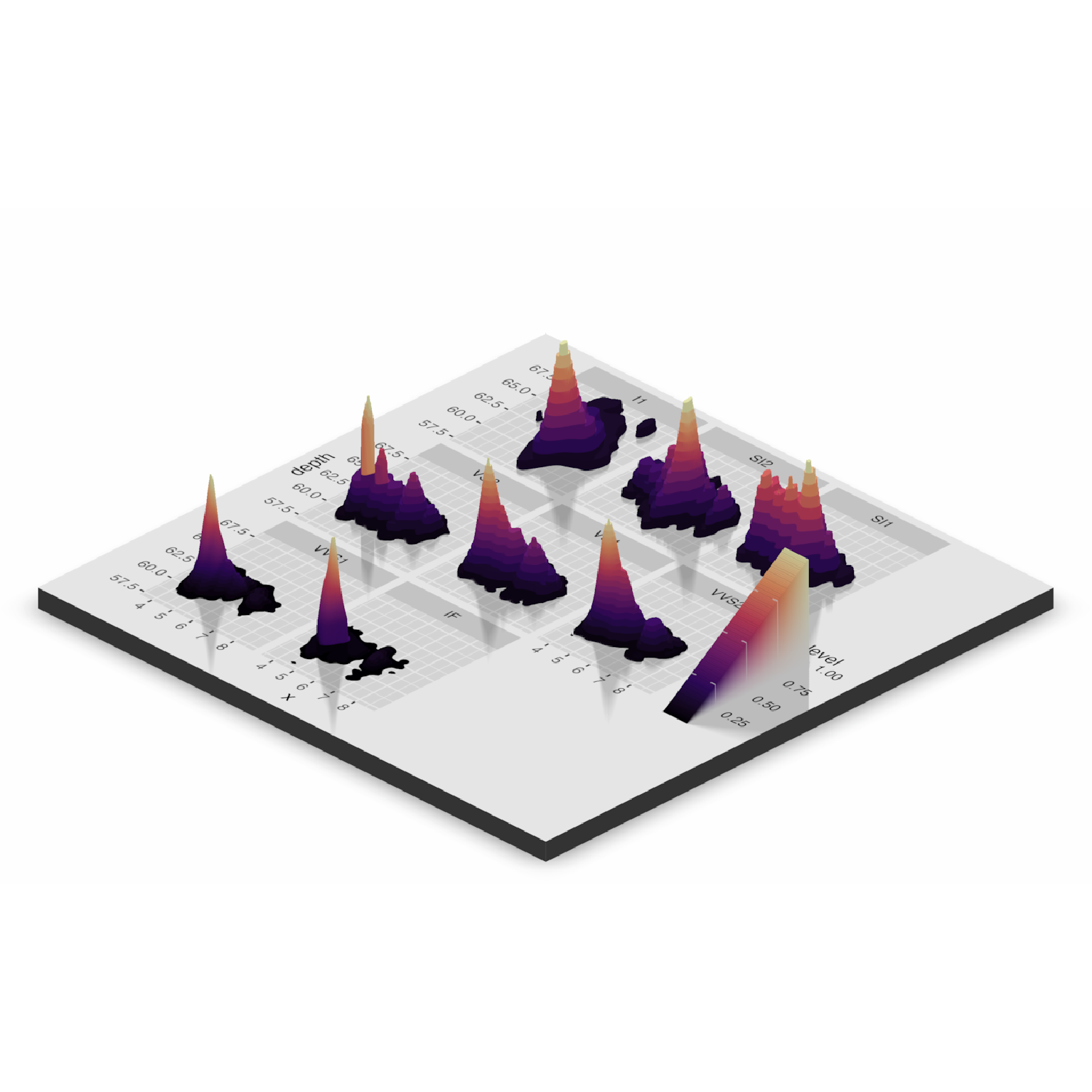# }
#Change the camera angle and take a snapshot:
# \dontrun{
render_camera(zoom=0.5,theta=-30,phi=30)
render_snapshot(clear = TRUE)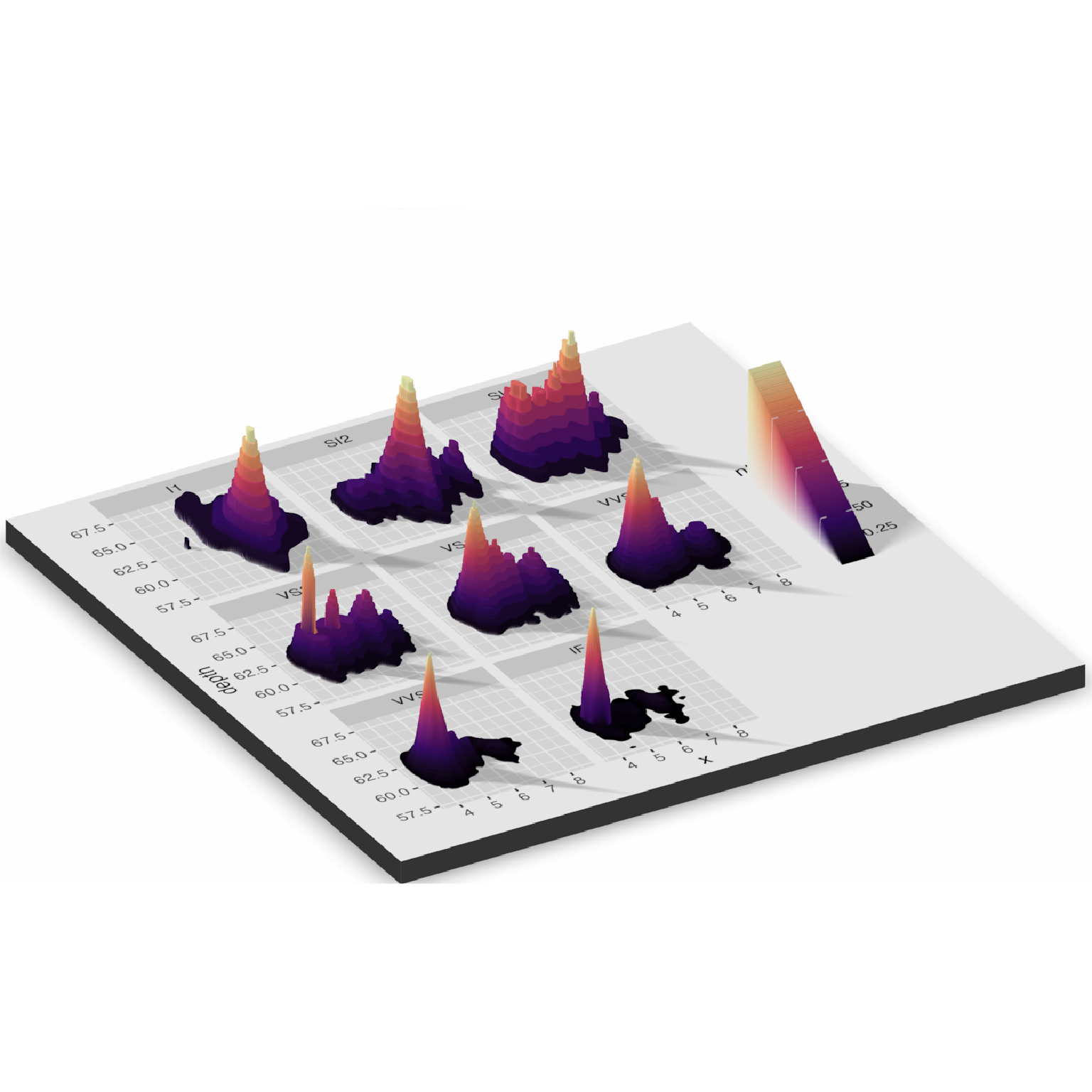# }

#Contours and other lines will automatically be ignored. Here is the volcano dataset:

ggvolcano = volcano %>%
reshape2::melt() %>%
ggplot() +
geom_tile(aes(x=Var1,y=Var2,fill=value)) +
geom_contour(aes(x=Var1,y=Var2,z=value),color="black") +
scale_x_continuous("X",expand = c(0,0)) +
scale_y_continuous("Y",expand = c(0,0)) +
coord_fixed()
ggvolcano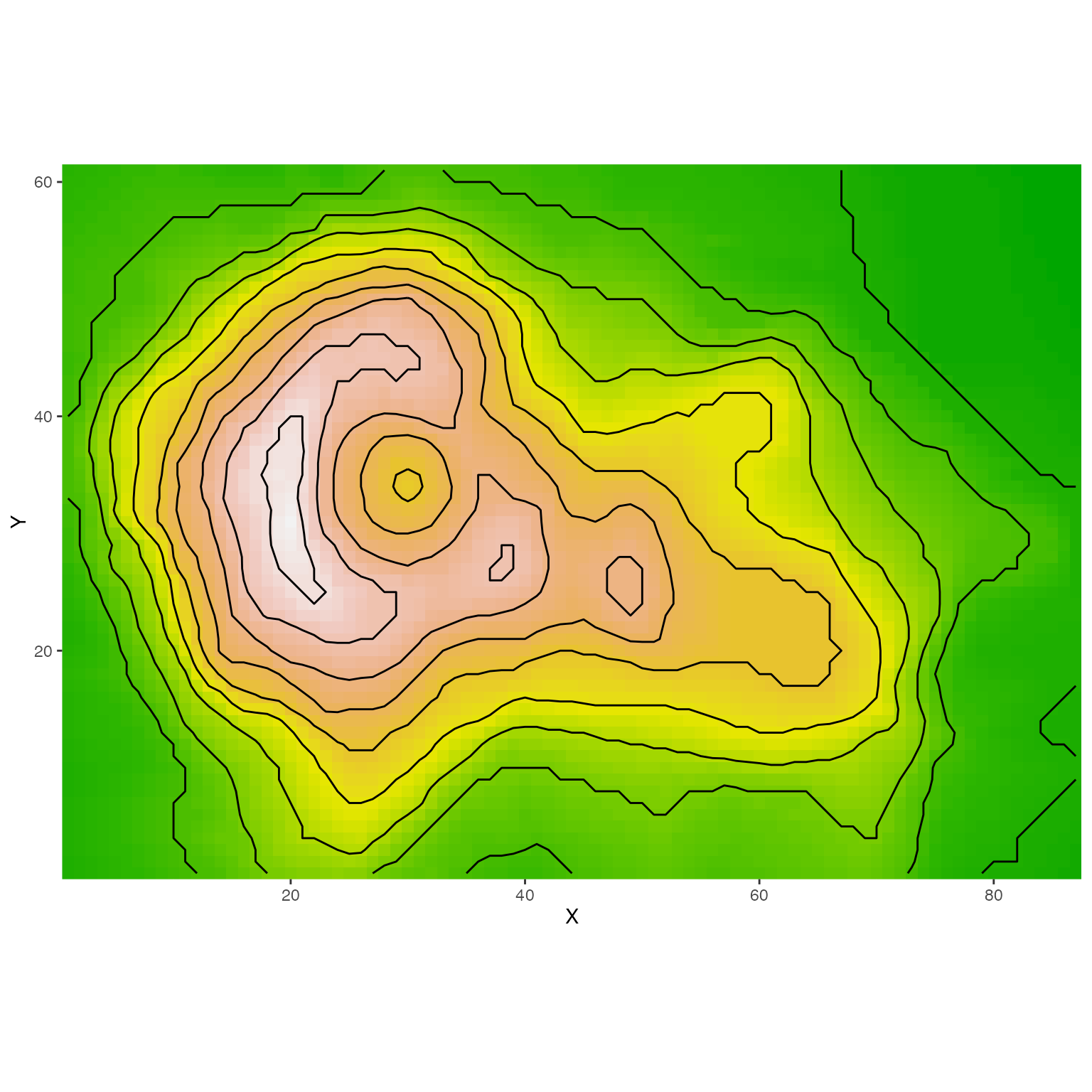# \dontrun{
plot_gg(ggvolcano, multicore = TRUE, raytrace = TRUE, width = 7, height = 4,
scale = 300, windowsize = c(1400, 866), zoom = 0.6, phi = 30, theta = 30)
#> Warning: Removed 1861 row(s) containing missing values (geom_path).
render_snapshot(clear = TRUE)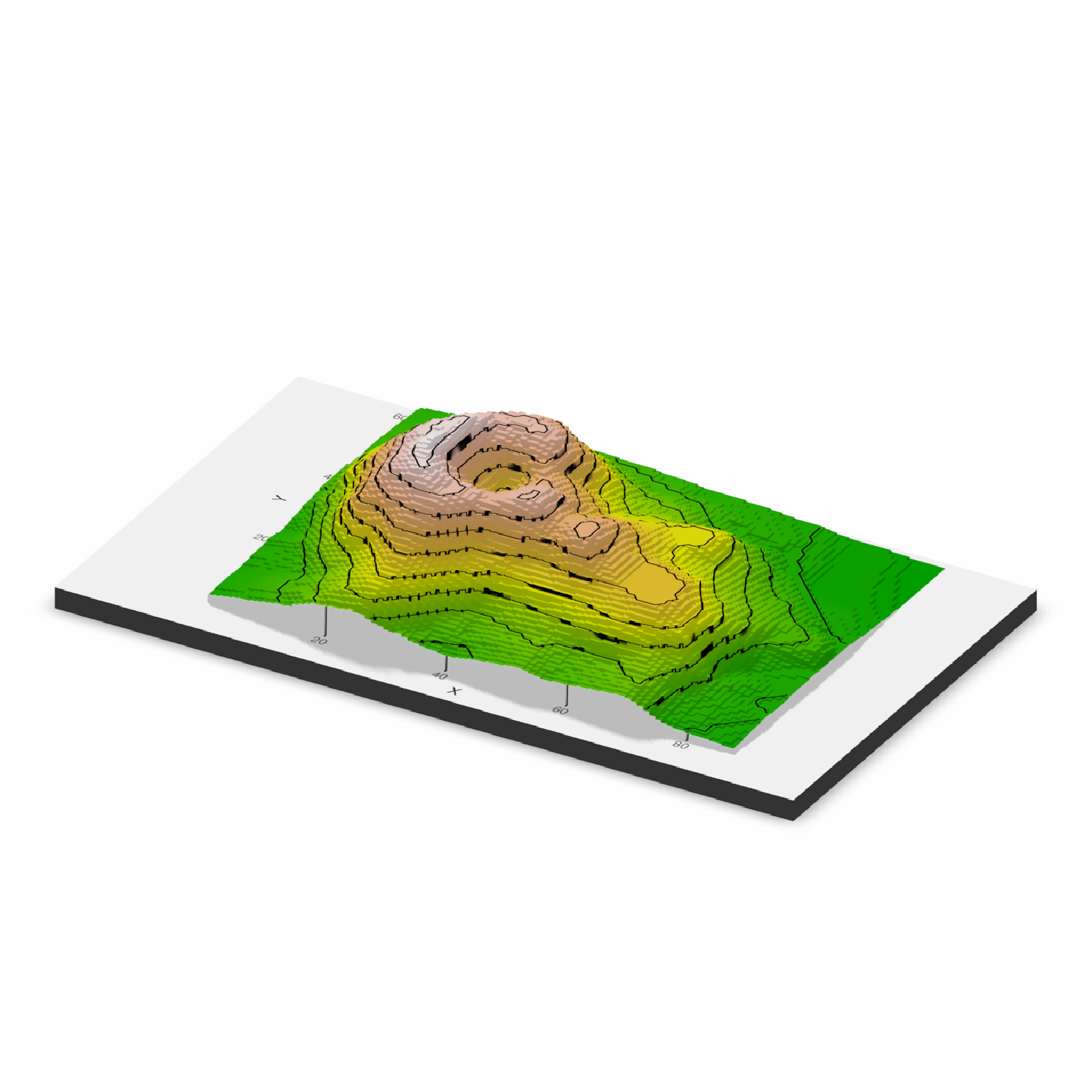# }
#Here, we will create a 3D plot of the mtcars dataset. This automatically detects
#that the user used the color aesthetic instead of the fill.
mtplot = ggplot(mtcars) +
geom_point(aes(x=mpg,y=disp,color=cyl)) +
scale_color_continuous(limits=c(0,8))

#Preview how the plot will look by setting preview = TRUE: We also adjust the angle of the light.
# \dontrun{
plot_gg(mtplot, width=3.5, sunangle=225, preview = TRUE)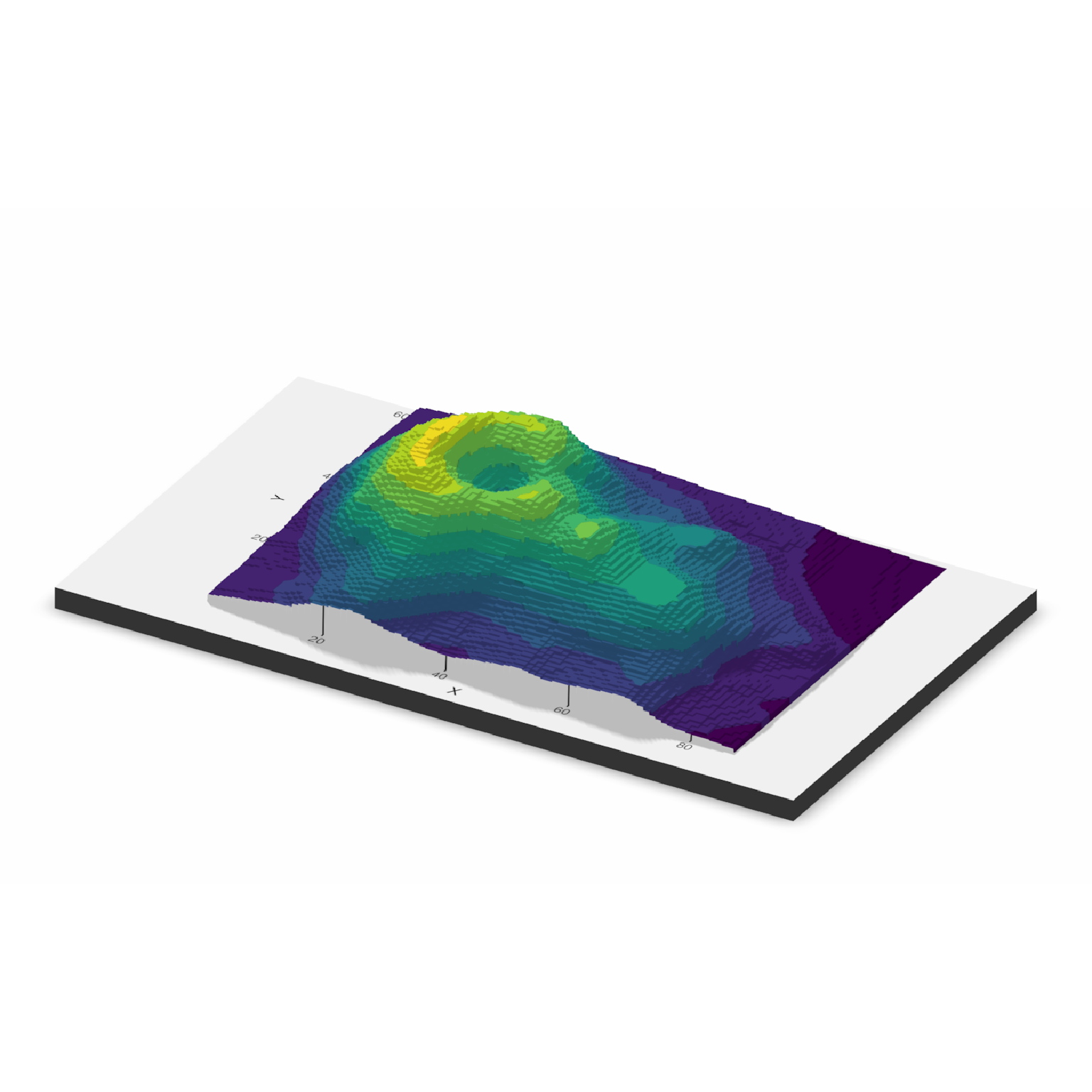# }
# \dontrun{
plot_gg(mtplot, width=3.5, multicore = TRUE, windowsize = c(1400,866), sunangle=225,
zoom = 0.60, phi = 30, theta = 45)
render_snapshot(clear = TRUE)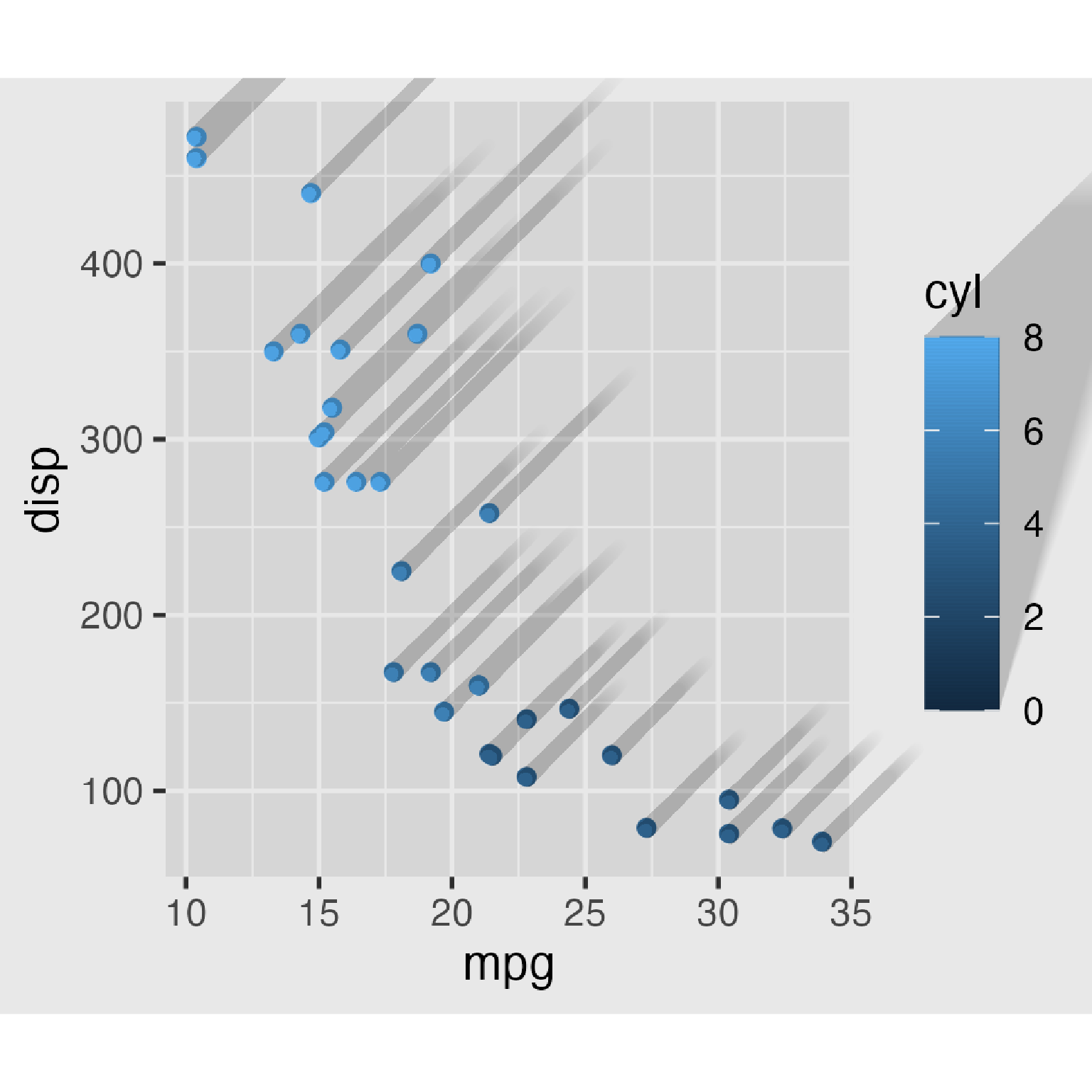# }
#Now let's plot a density plot in 3D.
mtplot_density = ggplot(mtcars) +
stat_density_2d(aes(x=mpg,y=disp, fill=..density..), geom = "raster", contour = FALSE) +
scale_x_continuous(expand=c(0,0)) +
scale_y_continuous(expand=c(0,0)) +
mtplot_density# \dontrun{
plot_gg(mtplot_density, width = 4,zoom = 0.60, theta = -45, phi = 30,
windowsize = c(1400,866))
render_snapshot(clear = TRUE)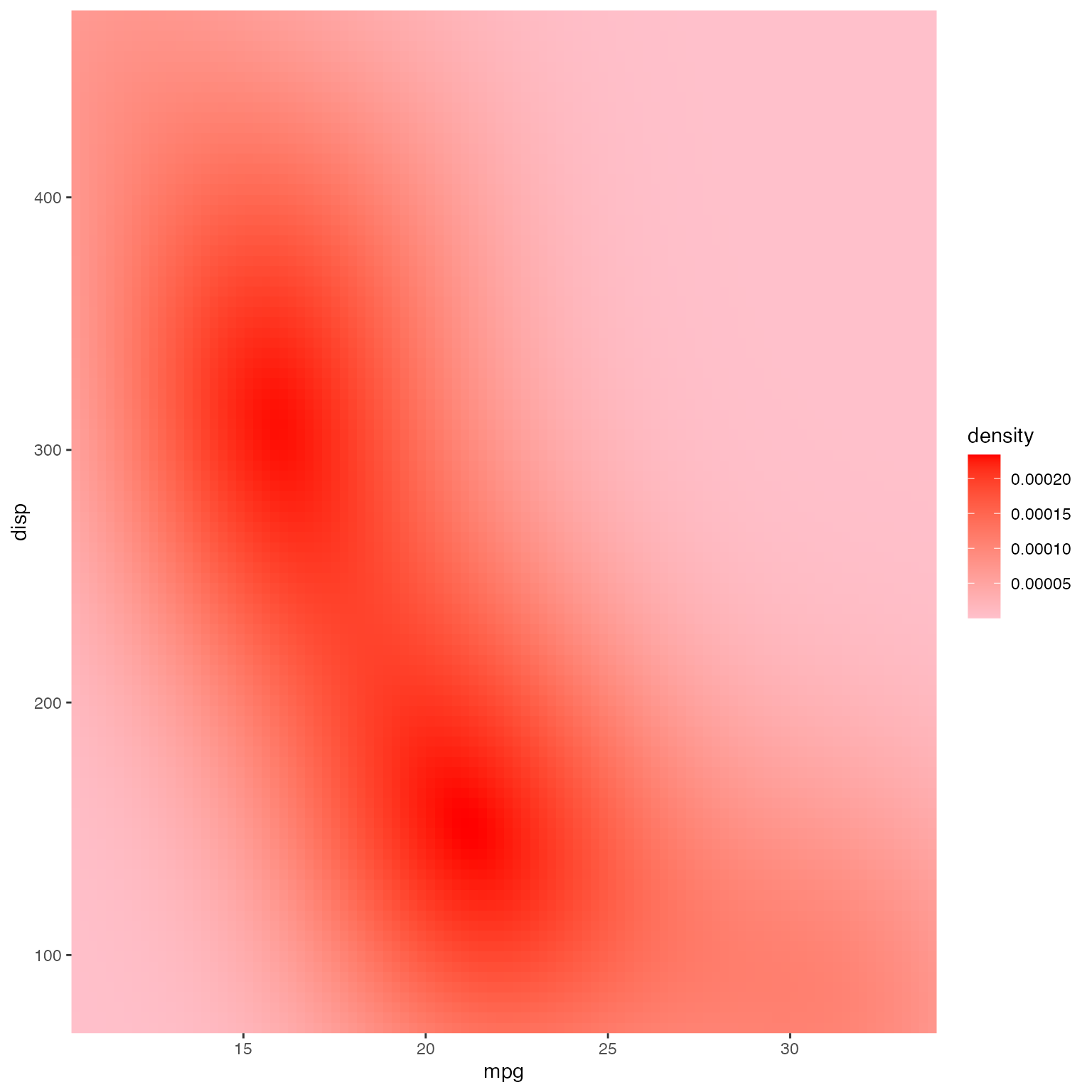# }
#This also works facetted.
mtplot_density_facet = mtplot_density + facet_wrap(~cyl)

#Preview this plot in 2D:
# \dontrun{
plot_gg(mtplot_density_facet, preview = TRUE)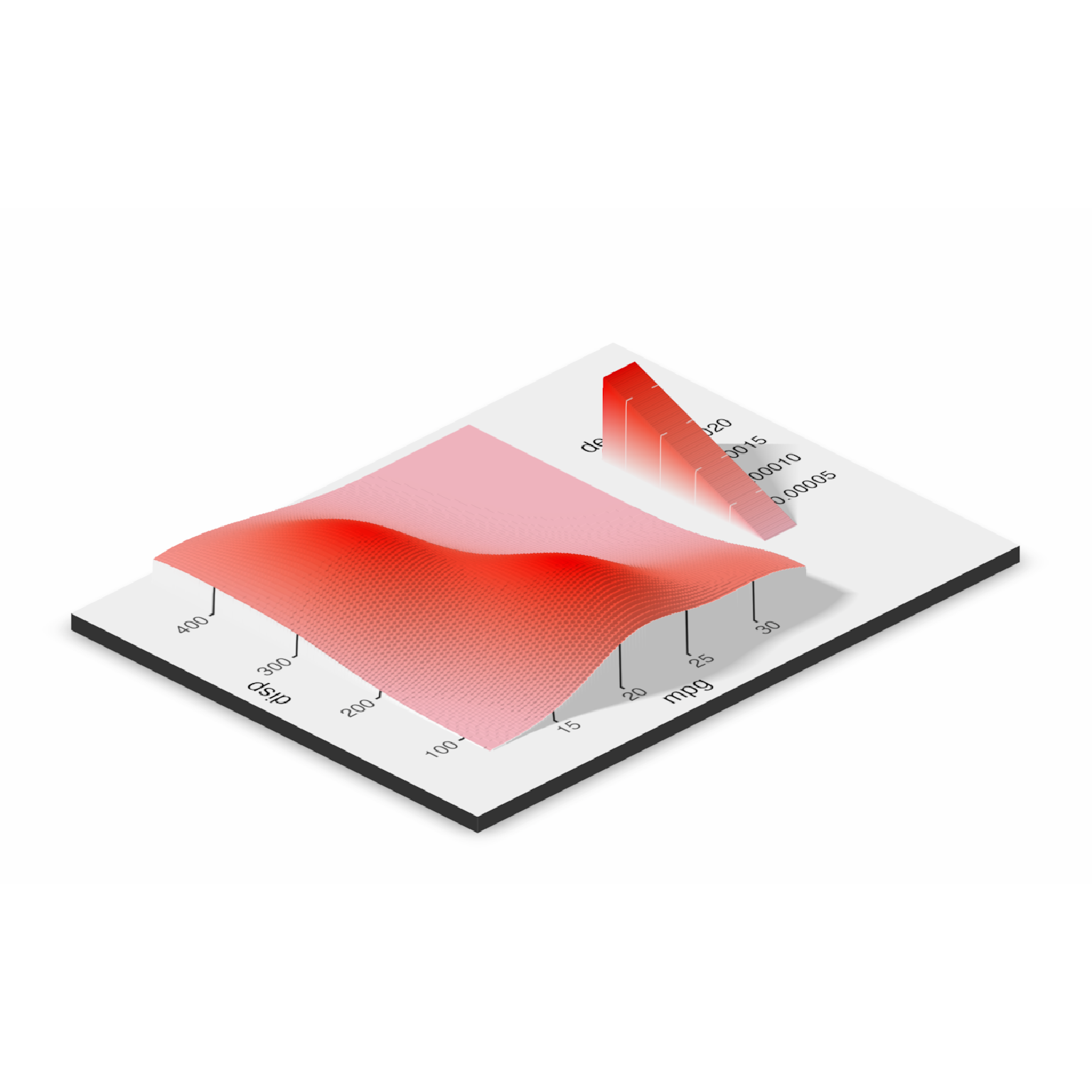# }
# \dontrun{
plot_gg(mtplot_density_facet, windowsize=c(1400,866),
zoom = 0.55, theta = -10, phi = 25)
render_snapshot(clear = TRUE)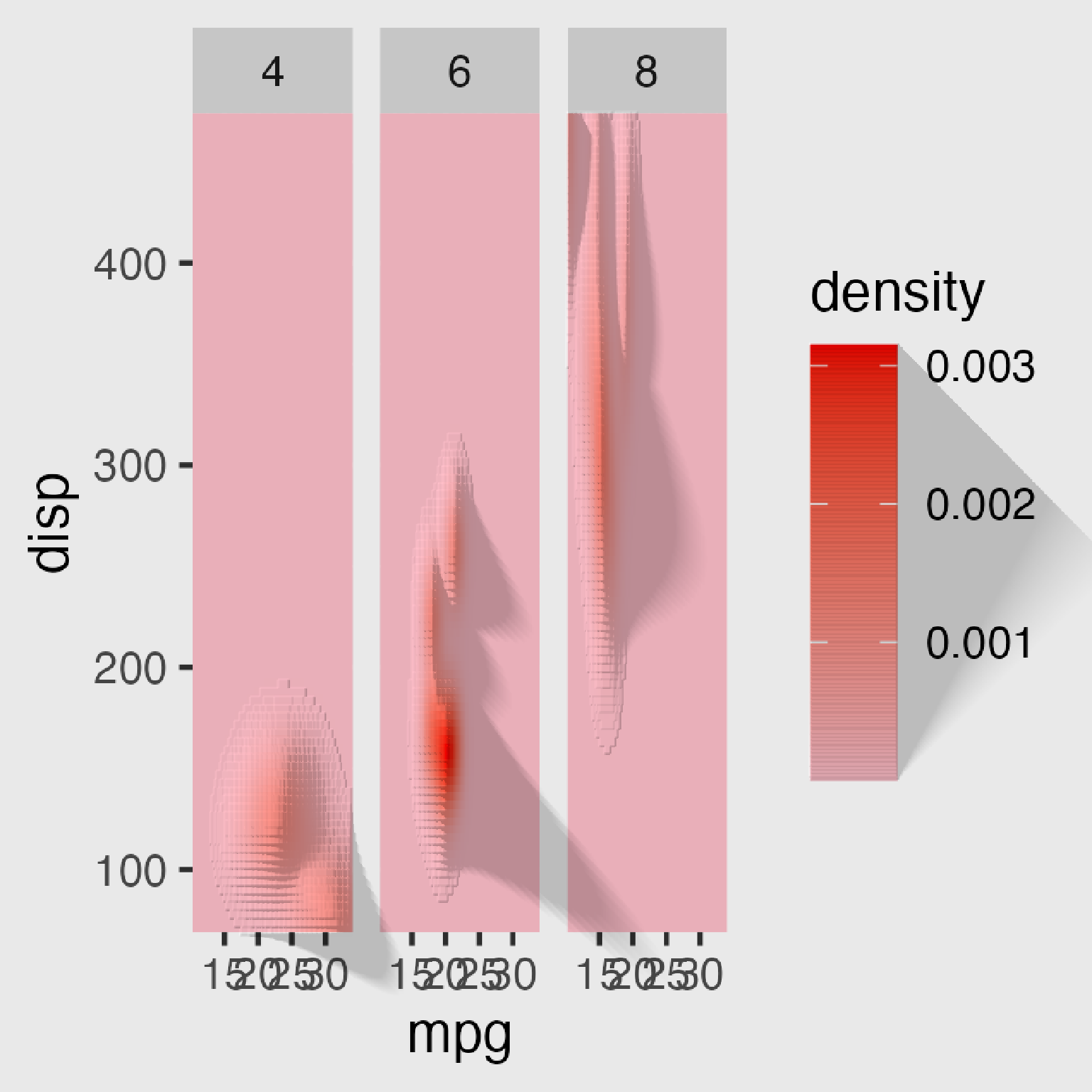# }
#That is a little cramped. Specifying a larger width will improve the readability of this plot.
# \dontrun{
plot_gg(mtplot_density_facet, width = 6, preview = TRUE)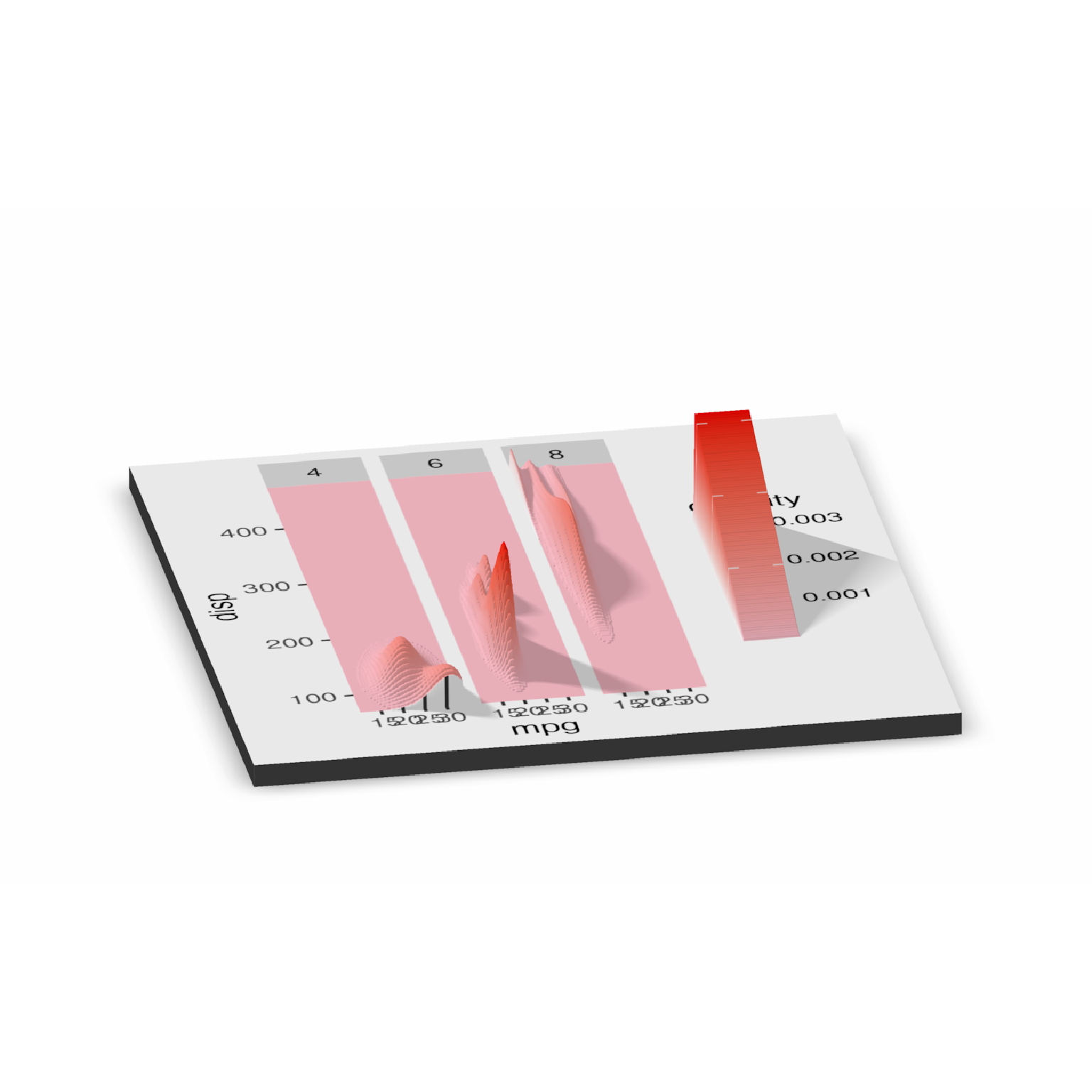# }

#That's better. Let's plot it in 3D, and increase the scale.
# \dontrun{
plot_gg(mtplot_density_facet, width = 6, windowsize=c(1400,866),
zoom = 0.55, theta = -10, phi = 25, scale=300)
render_snapshot(clear = TRUE)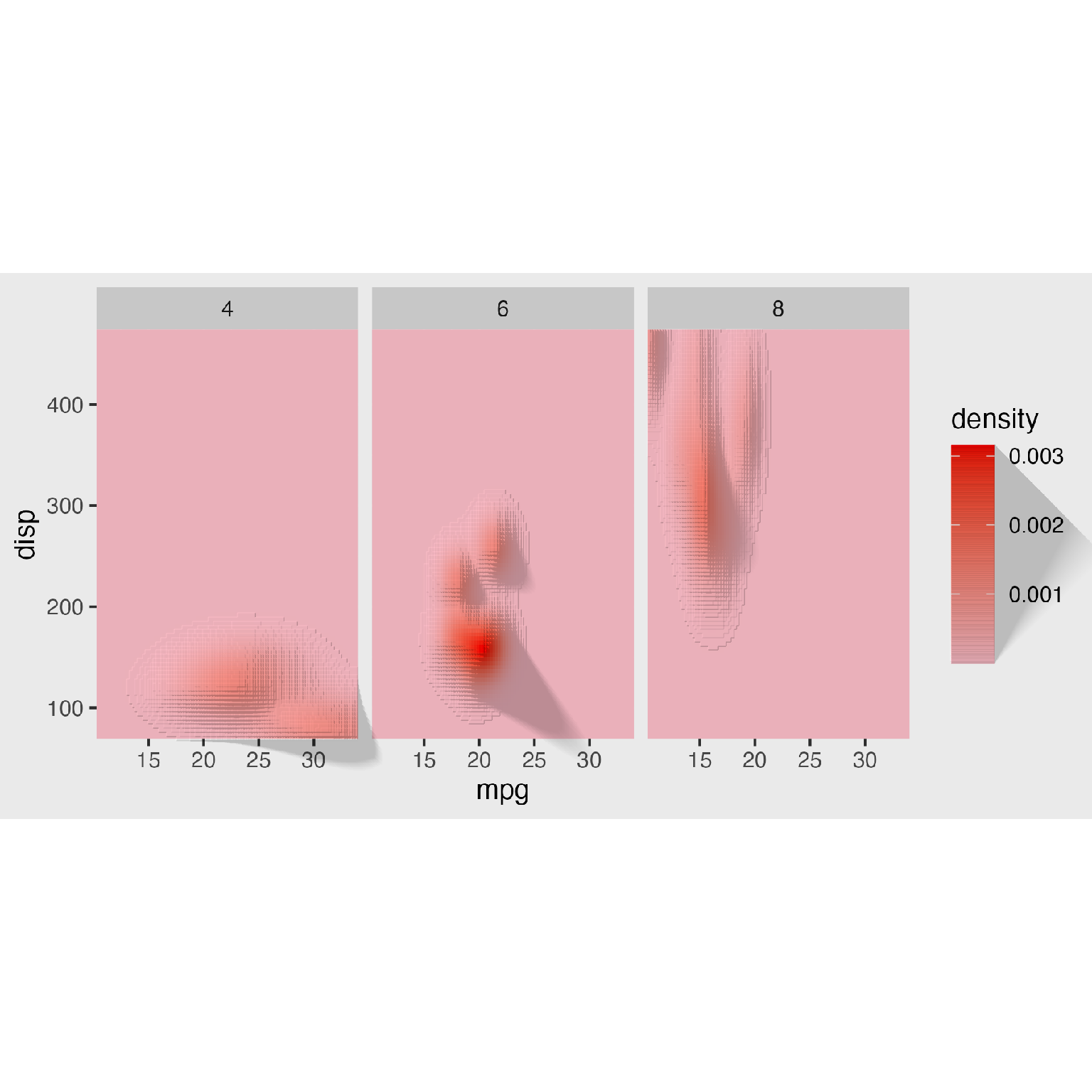# }
`